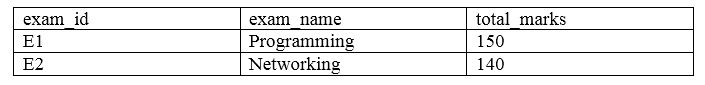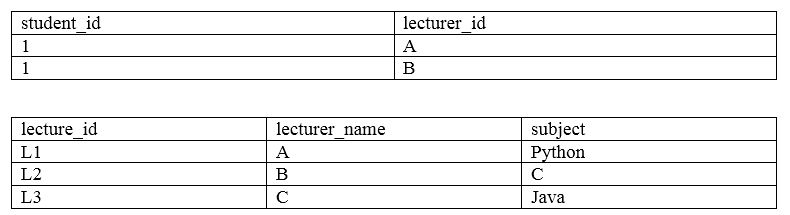# What is the Difference Between 3NF and BCNF

The main difference between 3NF and BCNF is that the table should be in 2nd normal form and there shouldn’t be any transitive dependencies to satisfy 3NF while the prime attributes of the table should not depend on the non-prime attributes of the table to satisfy BCNF.

Data redundancy refers to the same data repeating in multiple places. It can also cause issues in data insertion, update and delete. Normalization is the process of organizing data into multiple related tables in order to minimize data redundancy. It provides a solution to data redundancy as it divides data in a table into multiple tables. However, it is not possible to completely eliminate data redundancy; it is only possible to reduce data redundancy. There are various types of normalization such as 1NF, 2nf, 3NF, BCNF, etc.  Before discussing 3NF and BCNF, it is necessary to understand 1NF and 2NF.

### Key Areas Covered

1. What is 1NF
– Definition, Functionality
2. What is 2NF
– Definition, Functionality
3. What is 3NF
-Definition, Functionality
4. What is BCNF
-Definition, Functionality
5. What is the Difference Between 3NF and BCNF
-Comparison of Key Differences

### Key Terms

1NF, 2NF, 3NF, BCNF, Normalization## What is 1NF

There are four rules to make a table satisfy 1NF. They are as follows.

• Each column in the table should have automatic values.
• A column should have data of the same type.
• Each column should have a unique name.
• Order of storing data does not matter.

If a table is not in the 1st normal form, it is considered as a bad database design. An example is as follows.In the above table, the subject column contains multiple values. After performing 1NF, the table is as follows.## What is 2NF

There are two rules to make a table satisfy 2NF. They are as follows.

• The table should be in 1NF form.
• There should be no partial dependencies.

In a table that stores information of students, the student id is the primary key, and it helps to identify each row in the table separately. Other columns depend on the primary key. This dependency is called functional dependency.

For example, assume there is a table called score. The primary key is the combination of student id and subject id. If this table has lecturer name, it only depends on the subject id. This kind of dependency is called partial dependency.

An example is as follows.The primary key of the above table is the combination of student_id and subject_id. We can place the lecturer_name in a separate table. Now the score, subject and lecturer tables are as follows.## What is 3NF

There are two rules to make a table satisfy 2nf. They are as follows.

• The table should satisfy the 2nd normal form.
• It should not have transitive.For example, assume that the score table has student_id, subject_id, marks, exam_name and total_marks. The combination of student_id and subject_id is the primary key. This total_marks does not depend on this primary key. It depends on the exam_name. In other words, an attribute depends on the non-prime attribute. This kind of dependency is called transitive dependency. Therefore, we can score the exam-name and total-marks in a separate table.## What is BCNF

BCNF stands for Boyce-Codd-Normal Form. It avoids prime attributes depending on the non-prime attributes. An example is as follows.The primary key of the above table is the combination of student_id and subject. As one lecturer lectures one specific subject, we can also use that column to identify each record uniquely. Nevertheless, lecturer is not a prime key. The subject column depends on the non-prime attribute lecturer. Therefore, the table does not satisfy BCNF. We can create student table and lecturer table as follows.## Difference Between 3NF and BCNF

### Definition

3NF is a normal form that is used in normalizing a database design to reduce the duplication of data and ensure referential integrity by ensuring that the entity is in second normal form and all the attributes in a table are determined only by the candidate keys of that relation and not by any non-prime attributes. BCNF, on the other hand, is a normal form used in database normalization which is a slightly stronger version of the 3NF. Thus, this is the main difference between 3NF and BCNF.

### Functionality

The table should be in 2nd normal form, and there shouldn’t be any transitive dependencies to satisfy 3NF while the prime attributes of the table should not depend on the non-prime attributes of the table to satisfy BCNF. Hence, functionality is another difference between 3NF and BCNF.

### Conclusion

In brief, a normalized database is considered as a good database. There are different types of normalization, and two of them are 3NF and BCNF. The main difference between 3NF and BCNF is that the table should be in 2nd normal form and there shouldn’t be any transitive dependencies to satisfy 3NF while the prime attributes of the table should not depend on the non-prime attributes of the table to satisfy BCNF.

##### Reference:

1. First Normal Form (1NF) | Database Normalization | DBMS, Studytonight, 24 Dec. 2017, Available here.
2. Second Normal Form (2NF) | Database Normalization | DBMS, Studytonight, 4 Feb. 2018, Available here.
3. Third Normal Form (3NF) | Database Normalization | DBMS, Studytonight, 8 Feb. 2018, Available here.
4. Boyce-Codd Normal Form (BCNF) | Database Normalization | DBMS, Studytonight, 15 Apr. 2018, Available here.
5. “Third Normal Form.” Wikipedia, Wikimedia Foundation, 28 Nov. 2018, Available here.
6. “Boyce–Codd Normal Form.” Wikipedia, Wikimedia Foundation, 27 Sept. 2018, Available here.

##### Image Courtesy:

1. “1895779” (CC0) via Pixabay